## 机器学习：支持向量机实现与应用

8539 字 · 292 阅读 · 2023 年 05 月 10 日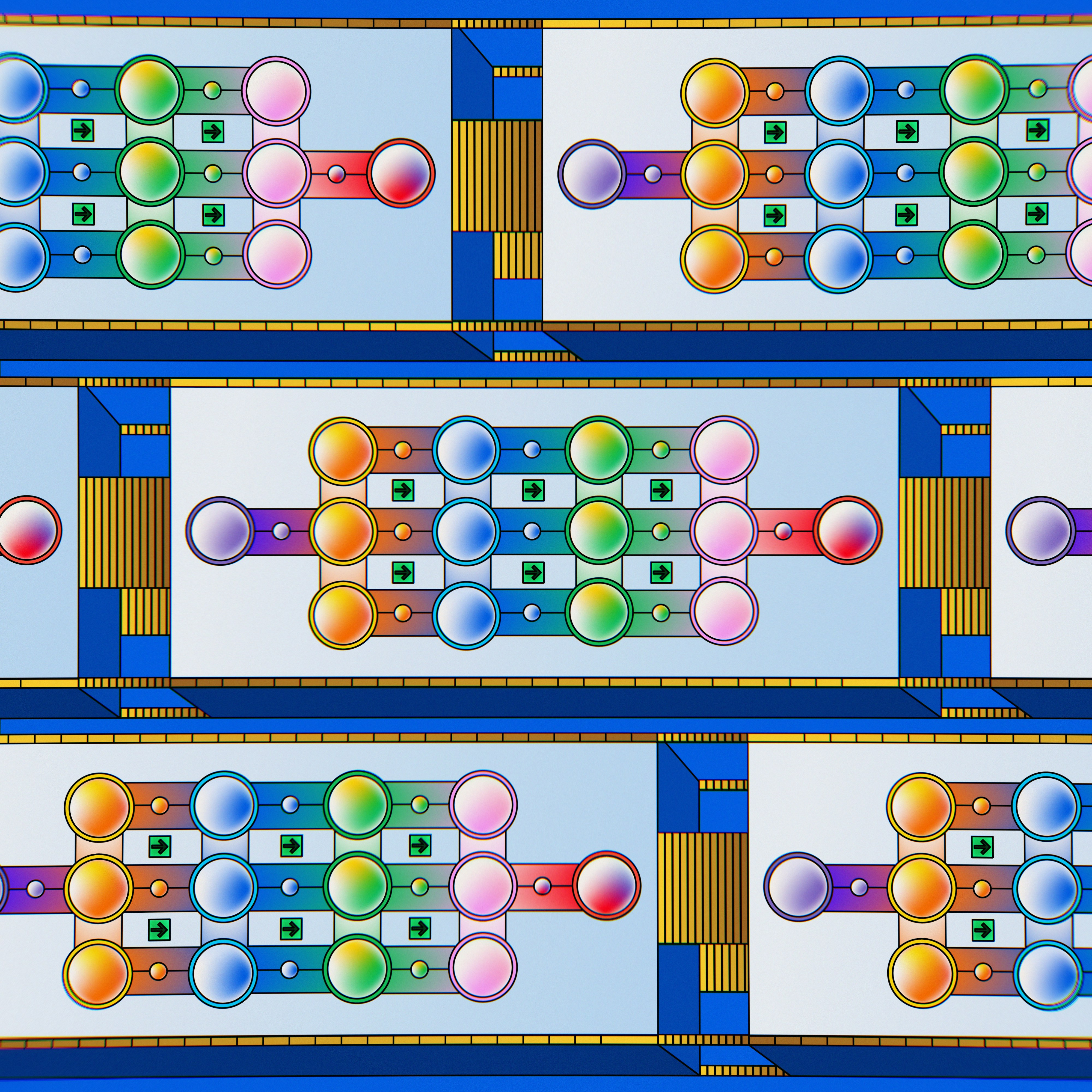• 线性分类支持向量机
• 拉格朗日对偶性
• 线性支持向量机分类实现
• 非线性分类支持向量机
• 核技巧与核函数

### 线性分类支持向量机### 支持向量机分类特点

$$wx+b=0$$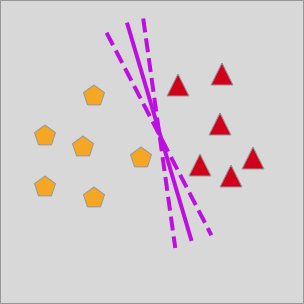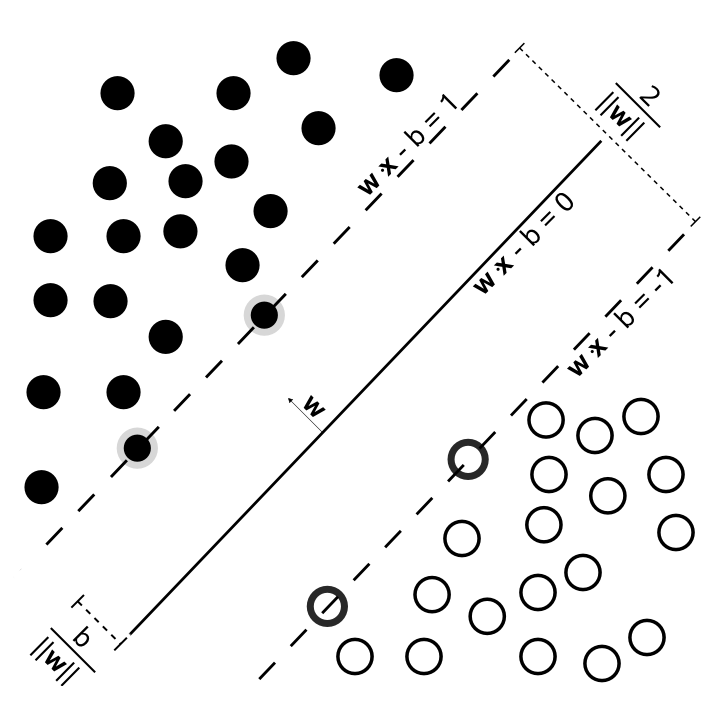### 支持向量机分类演示

from sklearn.datasets import samples_generator
import matplotlib.pyplot as plt
%matplotlib inline

x, y = samples_generator.make_blobs(
n_samples=60, centers=2, random_state=30, cluster_std=0.8)  # 生成示例数据

plt.figure(figsize=(10, 8))  # 绘图
plt.scatter(x[:, 0], x[:, 1], c=y, s=40, cmap='bwr')


import numpy as np

plt.figure(figsize=(10, 8))
plt.scatter(x[:, 0], x[:, 1], c=y, s=40, cmap='bwr')

# 绘制 3 条不同的分割线
x_temp = np.linspace(0, 6)
for m, b in [(1, -8), (0.5, -6.5), (-0.2, -4.25)]:
y_temp = m * x_temp + b
plt.plot(x_temp, y_temp, '-k')


plt.figure(figsize=(10, 8))
plt.scatter(x[:, 0], x[:, 1], c=y, s=40, cmap='bwr')

# 绘制 3 条不同的分割线
x_temp = np.linspace(0, 6)
for m, b, d in [(1, -8, 0.2), (0.5, -6.5, 0.55), (-0.2, -4.25, 0.75)]:
y_temp = m * x_temp + b
plt.plot(x_temp, y_temp, '-k')
plt.fill_between(x_temp, y_temp - d, y_temp +
d, color='#f3e17d', alpha=0.5)


### 函数间隔

$$h_{1} = y(w^{T}x +b) = yf(x) \tag{1}$$

$$h_{1} = min\: h_{1i}\: (i=1,2,...,n) \tag{2}$$

### 几何间隔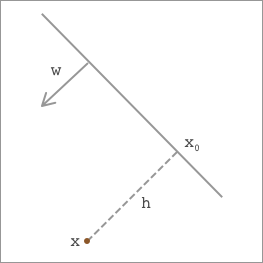$$x = x_{0}+h\frac{w}{\left \| w \right \|} \tag{3}$$

$$w ^ { T } x = w ^ { T } x _ { 0 } + h \frac { \| w \| ^ { 2 } } { \| w \| } \tag{4}$$

$$h = \frac{w^{T}x+b}{\left \| w \right \|}=\frac{f(x)}{\left \| w \right \|} \tag{5}$$

$$\left | h \right | = yh= \frac{h_{1}}{\left \| w \right \|} \tag{6}$$

### 拉格朗日对偶性

$$max\: \left | h \right |= max\: \frac{h_{1}}{\left \| w \right \|} \tag{7}$$

$$y_{i}(w^{T}x_{i}+b)\geq h_{1}\: \: (i=1,2,...,n) \tag{8}$$

$$max\: \frac{1}{\left \| w \right \|} \: \: \: \: s.t.\: \: y_{i}(w^{T}x_{i}+b)-1\geq 0\: \: (i=1,2,...,n) \tag{9}$$

$$min\: \frac{1}{2}\left \| w \right \|^{2} \: \: \: \: s.t.\: \: y_{i}(w^{T}x_{i}+b)-1\geq 0\: \: (i=1,2,...,n) \tag{10}$$

$$L(\lambda ,w,b)=\frac{1}{2}\left \| w \right \|^{2}-\sum_{i=1}^{n}\lambda_{i}(y_{i}(w^{T}x_{i}+b)-1) \tag{11}$$

$$\theta (w)=max\: L(\lambda ,w,b)\: \: \: \: \lambda_{i}\geq 0 \tag{12}$$

$$min_{(w,b)}\, max_{(\lambda \geq 0)}\, L(\lambda ,w,b) = p^{*} \tag{13}$$

$$max_{(\lambda \geq 0)}\, min_{(w,b)}\, L(\lambda ,w,b) = d^{*} \tag{14}$$

$$d^{*}=max_{(\lambda \geq 0)}\, min_{(w,b)}\, L(\lambda ,w,b)\leq min_{(w,b)}\, max_{(\lambda \geq 0)}\, L(\lambda ,w,b) = p^{*} \tag{15}$$

$$d^{*}=p^{*} \tag{16}$$

### 对偶问题求解

$$\bigtriangledown _{w}L(\lambda ,w,b)=w-\sum_{i=1}^{n}\lambda_{i}y_{i}x_{i}=0 \tag{17}$$
$$\bigtriangledown _{b}L(\lambda ,w,b) = \sum_{i=1}^{n}\lambda_{i}y_{i}=0 \tag{18}$$

$$L(\lambda ,w,b)=\sum_{i=1}^{n}\lambda_{i}-\frac{1}{2}\sum_{i,j=1}^{n}\lambda_{i}\lambda_{j}y_{i}y_{j}x_{i}^{T}x_{j} \tag{19}$$

$$max_{(\lambda )}\: \sum_{i=1}^{n}\lambda_{i}-\frac{1}{2}\sum_{i,j=1}^{n}\lambda_{i}\lambda_{j}y_{i}y_{j}x_{i}^{T}x_{j} \tag{20}$$
$$s.t.\: \:\sum_{i=1}^{n}\lambda_{i}y_{i}\: \: \: \: \lambda_{i}\geq 0,\: \: i=1,2,...,n$$

### 线性支持向量机分类实现

scikit-learn 中的支持向量机分类器对应的类及参数为：

sklearn.svm.SVC(C=1.0, kernel='rbf', degree=3, gamma='auto', coef0=0.0, shrinking=True, probability=False, tol=0.001, cache_size=200, class_weight=None, verbose=False, max_iter=-1, decision_function_shape='ovr', random_state=None)


• C: 支持向量机中对应的惩罚参数。
• kernel: 核函数，linear, poly, rbf, sigmoid, precomputed 可选，下文详细介绍。
• degree: poly 多项式核函数的指数。
• tol: 收敛停止的容许值。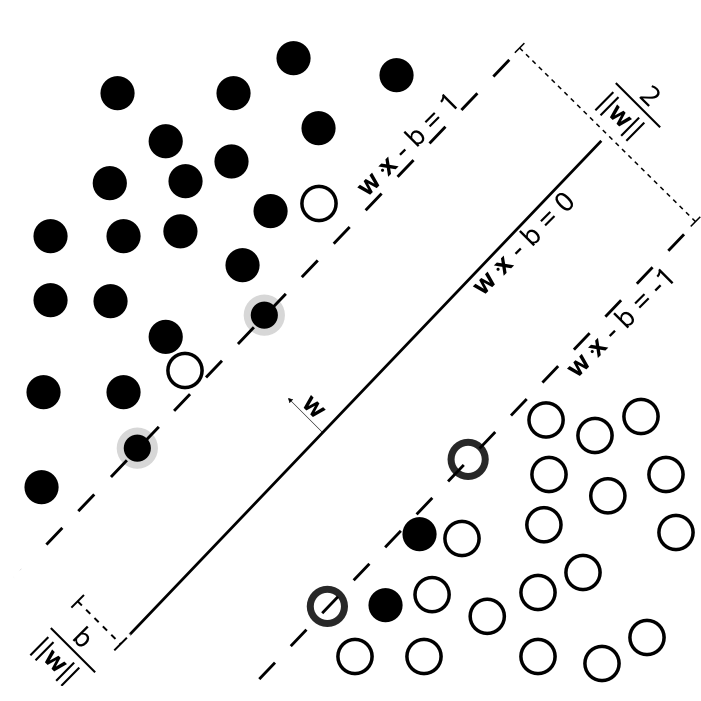from sklearn.svm import SVC

linear_svc = SVC(kernel='linear')
linear_svc.fit(x, y)


linear_svc.support_vectors_


def svc_plot(model):

# 获取到当前 Axes 子图数据，并为绘制分割线做准备
ax = plt.gca()
x = np.linspace(ax.get_xlim(), ax.get_xlim(), 50)
y = np.linspace(ax.get_ylim(), ax.get_ylim(), 50)
Y, X = np.meshgrid(y, x)
xy = np.vstack([X.ravel(), Y.ravel()]).T
P = model.decision_function(xy).reshape(X.shape)

# 使用轮廓线方法绘制分割线
ax.contour(X, Y, P, colors='green', levels=[-1, 0, 1], linestyles=['--', '-', '--'])

# 标记出支持向量的位置
ax.scatter(model.support_vectors_[:, 0], model.support_vectors_[:, 1], c='green', s=100)

# 绘制最大间隔支持向量图
plt.figure(figsize=(10, 8))
plt.scatter(x[:, 0], x[:, 1], c=y, s=40, cmap='bwr')
svc_plot(linear_svc)


# 向原数据集中加入噪声点
x = np.concatenate((x, np.array([[3, -4], [4, -3.8], [2.5, -6.3], [3.3, -5.8]])))
y = np.concatenate((y, np.array([1, 1, 0, 0])))

plt.figure(figsize=(10, 8))
plt.scatter(x[:, 0], x[:, 1], c=y, s=40, cmap='bwr')


linear_svc.fit(x, y) # 训练

plt.figure(figsize=(10, 8))
plt.scatter(x[:, 0], x[:, 1], c=y, s=40, cmap='bwr')
svc_plot(linear_svc)


from ipywidgets import interact
import ipywidgets as widgets

def change_c(c):
linear_svc.C = c
linear_svc.fit(x, y)
plt.figure(figsize=(10, 8))
plt.scatter(x[:, 0], x[:, 1], c=y, s=40, cmap='bwr')
svc_plot(linear_svc)

interact(change_c, c=[1, 10000, 1000000])


### 核技巧与核函数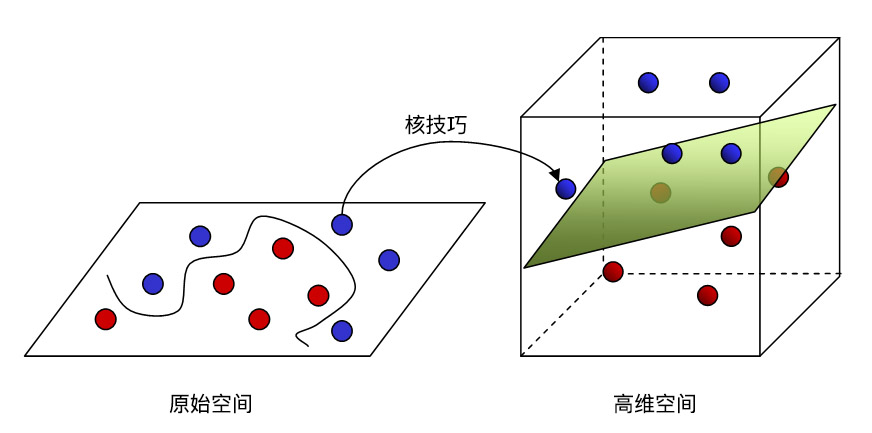$$k\left ( x_i, x_j \right )=x_i*x_j \tag{21}$$

$$k\left ( x_i, x_j \right )=\left ( x_i*x_j \right )^d, d \geq 1\tag{22}$$

$$k\left(x_{i}, x_{j}\right)=\exp \left(-\frac{\left\|\mathbf{x}_{\mathrm{i}}-\mathbf{x}_{\mathrm{j}}\right\|_{2}^{2}}{2 \sigma^{2}}\right)=\exp \left(-\gamma *\left\|x_{i}-x_{j}\right\|_{2}^{2}\right), \gamma>0 \tag{23}$$

Sigmoid 核函数：

$$k\left(x_{i}, x_{j}\right)=\tanh \left(\beta * x_{i} x_{j}+\theta\right), \beta>0, \theta<0 \tag{24}$$

$$\lambda_1 k_1+\lambda_2 k_2 \tag{25}$$

### 引入核函数的间隔表示及求解

$$\max\limits_{\lambda } \sum\limits_{i=1}^{N}\lambda _i - \frac{1}{2}\sum\limits_{i=1}^{N}\sum\limits_{j=1}^{N}\lambda_i\lambda_jy_iy_jk(x_i*x_j) \tag{26}$$
$$s.t. \sum\limits_{i=1}^{N}\lambda_iy_i=0$$
$$0 \leq \lambda_i \leq C ,i=1,2,...,N$$

$$w^{*}x+b^{*}=0 \tag{27}$$

$$f(x)=sign(w^{*}x+b^{*}) \tag{28}$$

### 非线性支持向量机分类实现

x2, y2 = samples_generator.make_circles(150, factor=.5, noise=.1, random_state=30) # 生成示例数据

plt.figure(figsize=(8, 8)) # 绘图
plt.scatter(x2[:, 0], x2[:, 1], c=y2, s=40, cmap='bwr')


$$k\left ( x_i, x_j \right )=x_i^2 + x_j^2 \tag{29}$$
def kernel_function(xi, xj):
poly = xi**2 + xj**2
return poly

from mpl_toolkits import mplot3d
from ipywidgets import interact, fixed

r = kernel_function(x2[:,0], x2[:,1])
plt.figure(figsize=(10, 8))
ax = plt.subplot(projection='3d')
ax.scatter3D(x2[:, 0], x2[:, 1], r, c=y2, s=40, cmap='bwr')
ax.set_xlabel('x')
ax.set_ylabel('y')
ax.set_zlabel('r')


rbf_svc = SVC(kernel='rbf', gamma='auto')
rbf_svc.fit(x2, y2)

plt.figure(figsize=(8, 8))
plt.scatter(x2[:, 0], x2[:, 1], c=y2, s=40, cmap='bwr')

svc_plot(rbf_svc)


def change_c(c):
rbf_svc.C = c
rbf_svc.fit(x2, y2)
plt.figure(figsize=(8, 8))
plt.scatter(x2[:, 0], x2[:, 1], c=y2, s=40, cmap='bwr')
svc_plot(rbf_svc)

interact(change_c, c=[1, 100, 10000])


### 多分类支持向量机

• 一对多法：即训练时依次把某个类别的样本归为一类，剩余的样本归为另一类，这样 $k$ 个类别的样本就构造出了 $k$ 个支持向量机。
• 一对一法：即在任意两类样本之间构造一个支持向量机，因此 $k$ 个类别的样本就需要设计 $k(k-1) \div 2$ 个支持向量机。

• decision_function_shape='ovo'：代表一对一法。
• decision_function_shape='ovr'：代表一对多法。

### 系列文章•